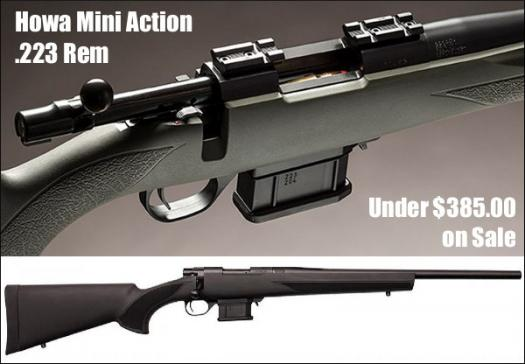# Do You Know The Varmint Gun?

10 Questions | Total Attempts: 105SettingsVarmint gun are guns also used for games. They have great velocity and are designed for varmint animals. On that note, how about some questions?

• 1.
Which of the following is also referred to as a varmint rifle?
• A.

Small caliber firearm

• B.

Large caliber firearm

• C.

All of the above

• D.

None of the above

• 2.
Which of the following is also referred to as a varmint rifle?
• A.

Low-powdered air gun

• B.

High-powdered air gun

• C.

Medium-powdered air gun

• D.

None of the above

• 3.
It uses a magnifying optics.
• A.

True

• B.

False

• C.

Maybe

• D.

I don't know

• 4.
It uses bullets of __________ size
• A.

Large

• B.

Small

• C.

All of the above

• D.

None of the above

• 5.
It is majorly used to attack which kind of animal?
• A.

Varmints

• B.

Venua

• C.

Large animals

• D.

None of the above

• 6.
What is the average velocity of modern varmint rifles?
• A.

310m/s

• B.

510m/s

• C.

910m/s

• D.

300m/s

• 7.
What is the average velocity of modern varmint rifles?
• A.

1000ft/s

• B.

8000ft/s

• C.

3000ft/s

• D.

None of the above

• 8.
What is the average velocity of .220 swift?
• A.

910m/s

• B.

1200m/s

• C.

800m/s

• D.

None of the above

• 9.
What is the average velocity of .220 swift?
• A.

1000ft/s

• B.

2000ft/s

• C.

3000ft/s

• D.

4000ft/s

• 10.
What is the average velocity of .204 swift?
• A.

1000m/s

• B.

600m/s

• C.

1200m/s

• D.

1500m/s

Related TopicsBack to top